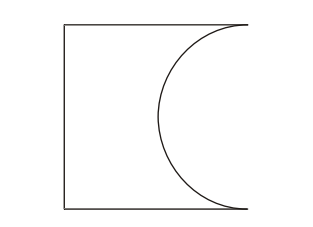# IMAT 2011 Q80 [Semicircular]

A square piece of metal has a semicircular piece cut out of it as shown. The area of the remaining metal is {100cm^2}.Which of the following is a correct expression of the length of the side of the square in cm?

A. 10 \sqrt{\frac {1}{8-π}}

B. 10 \sqrt{\frac {2}{4-π}}

C. 20 \sqrt{\frac{2}{8+π}}

D. 20 \sqrt{\frac{2}{8-π}}

E. 20 \sqrt{\frac{1}{4-π}}

When solving this types of questions the first thing you do is calculate the area of the main object (in this case, the square piece of metal)

In solving the question i will use b as a variable.

The area of the square is P_s=b^2.

A circle is defined by its radius. In this particular question, we can see that the radius is half of the length of the square edge, r=\frac{b}{2}.

The area of the circle is calculated by the formula: P_c=\pi r^2 Having seen previously what the value of r is we can get the final form of the area of the circle P_c=\pi \frac{b}{2}^2 \to P_c=\pi \frac{b^2}{4}.

In the area of the task, the semicircular piece cut out is actually half of the area of the circle we previously calculated.

So, in order to get the equation of the area of the remaining metal, we will subtract the area of half a circle from the square metal area: P = P_s - \frac{P_c}{2} \to P = b^2 - \frac{\pi\frac{b^2}{4}}{2} = b^2 - \frac{\pi b^2}{8} = b^2(1-\frac{\pi}{8}) = \frac{b^2}{8}(8-\pi) .

Using the value of the area given in the task we get an equation: P=\frac{b^2}{8}(8-\pi) =100cm^2. Multiplying the whole equation by 8 we get: b^2(8-\pi) =800cm^2 \to b^2 = \frac{800cm^2}{8-\pi} \to b=\sqrt{\frac{800cm^2}{8-\pi}} = 20\sqrt{\frac{2}{8-\pi}}cm.
Which is the solution to this question.